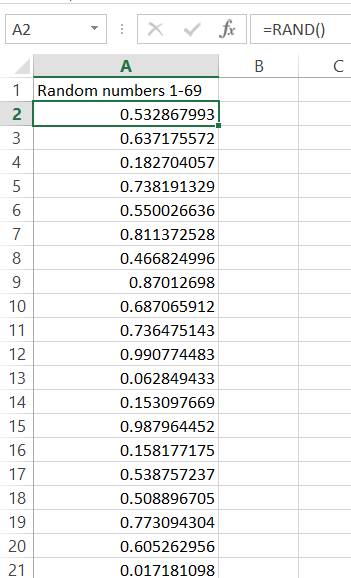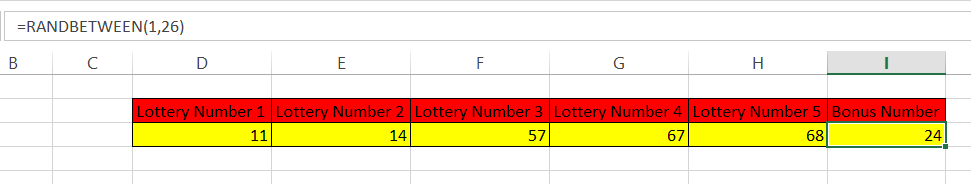### Lottery Number Generator in Excel

In this Excel lesson you will teach yourself how to prepare lottery number generator sheet in Excel.

### How to generate Random Numbers without Repeats?

Let’s suppose you would like to generate 5 different random numbers from 1 to 69.

To do that in Column A let’s use =RAND() Excel formula.Rand Excel function is doing just one simple thing. It is generating the random number. This is exactly what you need here! Let’s scroll down this to have exactly 69 same cells (you can choose different number, it’s is up to your needs. Lottery Number Generator will work for you. No worries!).

Now the question is.

How to draw the random number from that? You need to create a random numbers list without repeats.

Imagine the simplicity of the trick. You will generate 69 random numbers and to pick one just pick 5 rows which generated the largest numbers.

Isn’t it clever?

To pick the first random number just use this Excel formula:

=MATCH(LARGE(\$A\$2:\$A\$70,1),\$A\$2:\$A\$70,0)

It will generate the first random number. If it generated 7 it means that the 7th row generated the largest number. You can check that if you like.To generate more just change the digit 1 into next ones =MATCH(LARGE(\$A\$2:\$A\$70,2),\$A\$2:\$A\$70,0)

for second number,

=MATCH(LARGE(\$A\$2:\$A\$70,3),\$A\$2:\$A\$70,0)

for the third distinct number and so on).### How to generate one random number?

Let’s generate more. We need one more bonus number between 1 and 26.

How to generate such number?

There is nothing easier than that with Excel. Excel does have dedicated function for that. Just use RANKBETWEEN function:

Excel formula is

=RANDBETWEEN(1,26)

As you probably guessed 1 is bottom number and 26 is top one.Here is how the whole Lottery Number Generator looks like:Of course this spreadsheet is just a tool and it is not a guarantee to win main prize. Probability for that remains the same unfortunately.

We wish you generated only lucky lottery numbers and won fortune with our random lottery number generator.

## Template

```Further reading: